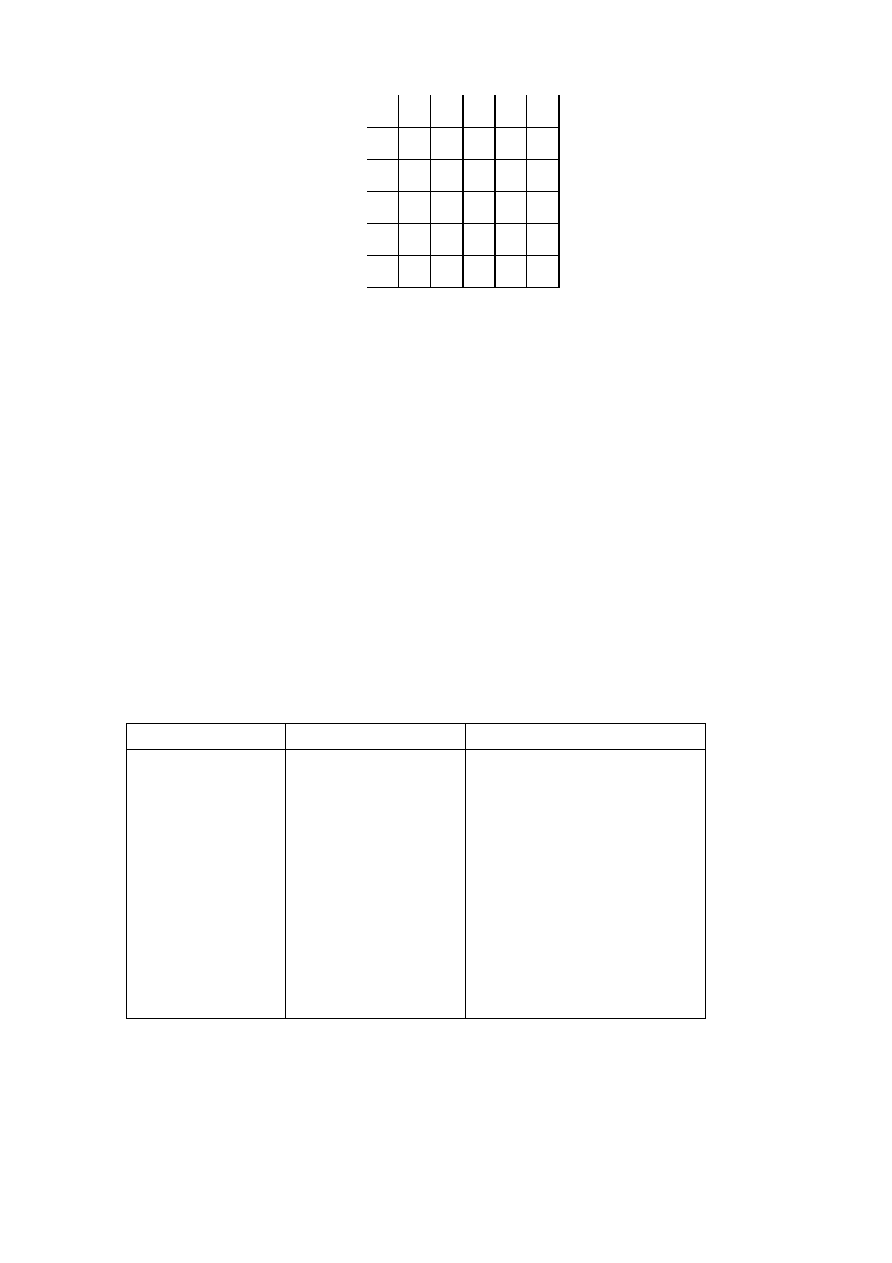ISO/IEC 10918-1 : 1993(E)
0
0
0
4
8
12
16
+S
+L
+S
­S
+L
­L
20
24
28
32
36
40
44
48
52
56
60
64
68
72
76
80
84
88
92
96
TISO1690-93/d107
­S
­L
Difference to left
(position a)
Difference above (position b)
Figure H.2 ­ 5 × 5 Conditioning array for two-dimensional statistical model
Figure H.2 [D107] = 7 cm = 273 %
H.1.2.3.2
Assignment of statistical bins to the DC binary
decision tree
Each statistics area for lossless coding consists of a contiguous set of 158 statistics bins. The first 100 bins consist of
25 sets of four bins selected by a context-index S0. The value of S0 is given by L_Context(Da,Db), which provides a
value of 0, 4,..., 92 or 96, depending on the difference classifications of Da and Db (see H.1.2.3.1). The value for S0
provided by L_Context(Da,Db) is from the array in Figure H.2.
The remaining 58 bins consist of two sets of 29 bins, X1, ..., X15, M2, ..., M15, which are used to code magnitude
category decisions and magnitude bits. The value of X1 is given by X1_Context(Db), which provides a value of 100 when
Db is in the zero, small positive or small negative categories and a value of 129 when Db is in the large positive or large
negative categories.
The assignment of statistical bins to the binary decision tree used for coding the difference is given in Table H.3.
Table H.3 ­ Statistical model for lossless coding
Context-index
Value
Coding decision
S0
L_Context(Da,Db)
V
=
0
SS
S0
+
1
Sign
SP
S0
+
2
Sz < 1 if V > 0
SN
S0
+
3
Sz < 1 if V < 0
X1
X1_Context(Db)
Sz < 2
X2
X1
+
1
Sz < 4
X3
X1
+
2
Sz < 8
.
.
.
.
.
.
X15
X1
+
14
Sz < 2
15
M2
X2
+
14
Magnitude bits if Sz < 4
M3
X3
+
14
Magnitude bits if Sz < 8
.
.
.
.
.
.
M15
X15
+
14
Magnitude bits if Sz < 2
15
CCITT Rec. T.81 (1992 E)
135